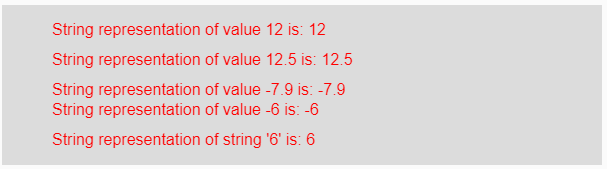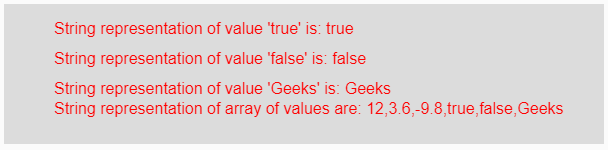# p5.js | str() function

The str() function in p5.js is used to convert the given boolean, string and number value into its string representation.

Syntax:

`str(value)`

Parameters: This function accepts single parameter value which is to be converted into its string representation. This value might be integer, float, string, boolean, negative or positive value and array of values.

Return Value: It returns the converted string representation.

Below programs illustrate the str() function in p5.js:

Example 1: This example uses str() function to convert the given input value into its corresponding string representation.

 `function` `setup() {  ` `  `  `    ``// Creating Canvas size ` `    ``createCanvas(600, 160);  ` `}  ` `  `  `function` `draw() {  ` `      `  `    ``// Set the background color  ` `    ``background(220);  ` `    `  `    ``// Initializing some values ` `    ``let Value1 = 12; ` `    ``let Value2 = 12.5; ` `    ``let Value3 = -7.9; ` `    ``let Value4 = -6; ` `    ``let Value5 = ``"6"``; ` `    `  `    ``// Calling to str() function. ` `    ``let A = str(Value1); ` `    ``let B = str(Value2); ` `    ``let C = str(Value3); ` `    ``let D = str(Value4); ` `    ``let E = str(Value5); ` `      `  `    ``// Set the size of text  ` `    ``textSize(16);  ` `      `  `    ``// Set the text color  ` `    ``fill(color(``'red'``));  ` `    `  `    ``// Getting string representation ` `    ``text(``"String representation of value 12 is: "` `+ A, 50, 30); ` `    ``text(``"String representation of value 12.5 is: "` `+ B, 50, 60); ` `    ``text(``"String representation of value -7.9 is: "` `+ C, 50, 90); ` `    ``text(``"String representation of value -6 is: "` `+ D, 50, 110); ` `    ``text(``"String representation of string '6' is: "` `+ E, 50, 140); ` `}  `

Output:Example 2: This example uses str() function to convert the given input value into its corresponding string representation.

 `function` `setup() {  ` `  `  `    ``// Creating Canvas size ` `    ``createCanvas(600, 140);  ` `}  ` `  `  `function` `draw() {  ` `      `  `    ``// Set the background color  ` `    ``background(220);  ` `    `  `    ``// Initializing some values ` `    ``let Value1 = ``true``; ` `    ``let Value2 = ``false``; ` `    ``let Value3 = ``"Geeks"``; ` `    ``let Value4 = [12, 3.6, -9.8, ``true``, ``false``, ``"Geeks"``]; ` `    `  `    ``// Calling to str() function. ` `    ``let A = str(Value1); ` `    ``let B = str(Value2); ` `    ``let C = str(Value3); ` `    ``let D = str(Value4); ` `      `  `    ``// Set the size of text  ` `    ``textSize(16);  ` `      `  `    ``// Set the text color  ` `    ``fill(color(``'red'``));  ` `    `  `    ``// Getting string representation ` `    ``text(``"String representation of value 'true' is: "` `+ A, 50, 30); ` `    ``text(``"String representation of value 'false' is: "` `+ B, 50, 60); ` `    ``text(``"String representation of value 'Geeks' is: "` `+ C, 50, 90); ` `    ``text(``"String representation of array of values are: "` `+ D, 50, 110); ` `}   `

Output:Reference: https://p5js.org/reference/#/p5/strMy Personal Notes arrow_drop_upCheck out this Author's contributed articles.

If you like GeeksforGeeks and would like to contribute, you can also write an article using contribute.geeksforgeeks.org or mail your article to contribute@geeksforgeeks.org. See your article appearing on the GeeksforGeeks main page and help other Geeks.

Please Improve this article if you find anything incorrect by clicking on the "Improve Article" button below.

Article Tags :

Be the First to upvote.

Please write to us at contribute@geeksforgeeks.org to report any issue with the above content.